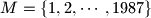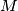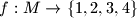### IMO Shortlist 1987 problem 17

Kvaliteta:
Avg: 0,0
Težina:
Avg: 0,0
Prove that there exists a four-coloring of the set$M = \{1, 2, \cdots, 1987\}$ such that any arithmetic progression with$10$ terms in the set$M$ is not monochromatic.

Alternative formulation

Let$M = \{1, 2, \cdots, 1987\}$. Prove that there is a function$f : M \to \{1, 2, 3, 4\}$ that is not constant on every set of$10$ terms from$M$ that form an arithmetic progression.

Proposed by Romania
Izvor: Međunarodna matematička olimpijada, shortlist 1987